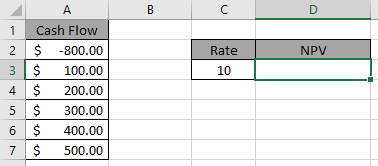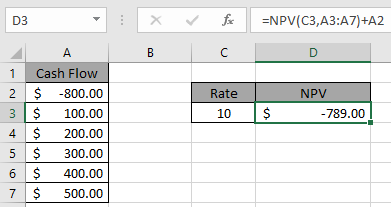# How to use the NPV function in Excel

In this article, we will learn about how to use NPV function in excel with formula.

NPV function is used to find the net present value of the data set in Excel.

Syntax:

=NPV(rate, value1, value2, ….)

Let’s use it in an example to understand it.Here one amount is debited and other amounts are credited at an interest of 10%.

Use the formula in D3 cell.

=NPV(C3, A3:A7)+A2Press Enter.It means the net present value of the whole expense is \$789.
This amount is to be debited.

Hope you understood how to use NPV function to get the net present value of the data. Explore more articles on Accounting here. Please fill out your queries in the comment box below. We will help you.

Related Articles:

How to use XNPV function in Excel

How to use the Excel IPMT Function

How to Use Compound Interest Function in Excel

Popular Articles:

How to use the VLOOKUP Function in Excel

How to use the COUNTIF function in Excel 2016

How to Use SUMIF Function in Excel

Terms and Conditions of use

The applications/code on this site are distributed as is and without warranties or liability. In no event shall the owner of the copyrights, or the authors of the applications/code be liable for any loss of profit, any problems or any damage resulting from the use or evaluation of the applications/code.